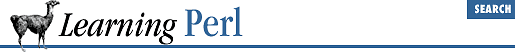home | O'Reilly's CD bookshelfs | FreeBSD | Linux | Cisco | Cisco Exam

### 2.7 <STDIN> as a Scalar Value

At this point, if you're a typical code hacker, you're probably wondering how to get a value into a Perl program. Here's the simplest way. Each time you use ``` <STDIN>``` in a place where a scalar value is expected, Perl reads the next complete text line from standard input (up to the first newline), and uses that string as the value of ``` <STDIN>``` . Standard input can mean many things, but unless you do something odd, it means the terminal of the user who invoked your program (probably you). If there's nothing waiting to be read (typically the case, unless you type ahead a complete line), the Perl program will stop and wait for you to enter some characters followed by a newline (return).

The string value of ``` <STDIN>``` typically has a newline on the end of it. Most often, you'll want to get rid of that newline right away (there's a big difference between ``` hello``` and ``` hello\n``` ). This is where our friend, the ``` chomp``` function, comes to the rescue. A typical input sequence goes something like this:

```\$a = <STDIN>;  # get the text

chomp(\$a);     # get rid of that pesky newline```

A common abbreviation for these two lines is:

`chomp(\$a = <STDIN>);`

The assignment inside the parentheses continues to refer to ``` \$a``` , even after it has been given a value with ``` <STDIN>``` . Thus, the ``` chomp``` function is working on ``` \$a``` . (This is true in general about the assignment operator; an assignment expression can be used wherever a variable is needed, and the actions refer to the variable on the left side of the equal sign.)2.6 Scalar Operators and Functions2.8 Output with print